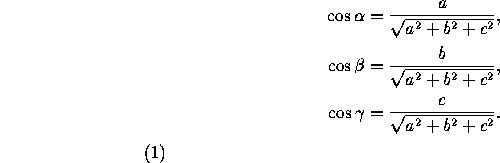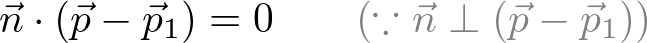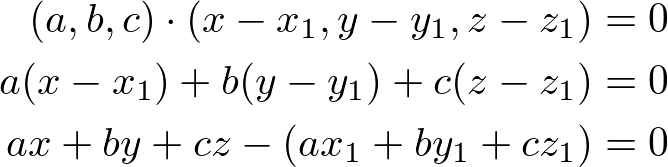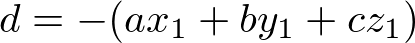# Unit-5: 3 D Co-ordinate Geometry

A three-dimensional Cartesian coordinate system is formed by a point called the origin (denoted by O) and a basis consisting of three mutually perpendicular vectors. … The coordinates of any point in space are determined by three real numbers: x, y, z.

### Coordinates in Space

Let there be a point P in space as shown in the figure below. If we drop a perpendicular PB on the XY plane and then from point B, we drop perpendiculars BA and BC on the x-axis and y-axis respectively. Assuming the length of the perpendiculars BC, BA and PB as x, y and z respectively. These lengths x, y and z are known as the co-ordinates of the point P in three-dimensional space. It must be noted that while giving the coordinates of a point, we always write them in order such that the co-ordinate of x-axis comes first, followed by the co-ordinate of the y-axis and the z-axis. Thus for each point in space there exist an ordered 3-tuple of real numbers for its representation.

### direcion angle and direction cosine

the direction cosines (or directional cosines) of a vector are the cosines of the angles between the vector and the three coordinate axes. Equivalently, they are the contributions of each component of the basis to a unit vector in that direction.

Given a vector (a,b,c) in three-space, the direction cosines of this vector areHere the direction angles , ,  are the angles that the vector makes with the positive x-, y– and z-axes, respectively. . We have

## Equation of line

Let us see see, how to derive the slope-intercept form equation of a straight through the following steps.

Step 1 :

Let L be a line with slope m and y-intercept b. Circle the point that must be on the line. Justify your choice.

(b, 0)   (0, b)   (0, m)   (m, 0)

The coordinate of x is 0 in the point that includes the y-intercept.

Step 2 :

Recall that slope is the ratio of change in y to change in x. Complete the equation for the slope m of the line using the y-intercept (0, b), and another point (x, y) on the line.

Slope m  =  change in y-values / change in x-values

Slope m  =  (y – b) / (x – 0)

Slope m  =  (y – b) / x

Step 3 :

In an equation of a line, we often want y by itself on one side of the equation. Solve the equation from Step 2 for y.

m  =  (y – b) / x

Multiply both sides by x

m.x  =  [(y – b) / x].x

mx  =  y – b

Add b to both sides of the equation.

mx + b  =  (y – b) + b

mx + b  =  y

Write the equation with y on the left side.

y  =  mx + b

## Shortest Distance Between Two Parallel Lines

Formula to find distance between two parallel line:

Consider two parallel lines are represented in the following form :

y = mx + c1 …(i)

y = mx + c2 ….(ii)

Where m = slope of line

Then, the formula for shortest distance can be written as under :

If the equations of two parallel lines are expressed in the following way :

ax + by + d1 = 0

ax + by + d2 = 0

then there is a little change in the formula.

#### shortest distance between two skew lines

Skew Lines are basically, lines that neither intersect each other nor are they parallel to each other in the three-dimensional space.The shortest distance between skew lines is equal to the length of the perpendicular between the two lines

Note that in case the two skew lines are intersecting, the shortest distance between them must necessarily be zero

### Plane

plane in space is defined by three points (which don’t all lie on the same line) or by a point and a normal vector to the plane.

#### Equation of plane

The equation of a plane in 3D space is defined with normal vector (perpendicular to the plane) and a known point on the plane.

Let the normal vector of a plane,and the known point on the plane, P1. And, let any point on the plane as P.

We can define a vector connecting from P1 to P, which is lying on the plane.Since the vectorand the normal vectorare perpendicular each other, the dot product of two vector should be 0.This dot product of the normal vector and a vector on the plane becomes the equation of the plane. By calculating the dot product, we get;If we substitute the constant terms to, then the plane equation becomes simpler;#### condition for a line to lie in a plane

We observe that a straight line will lie in a plane if every point on the line, lie in the plane and the normal to the plane is perpendicular to the line.

i) If the linelies in the plane, then⋅d and.= 0

ii) if the linelies in the plane Ax By Cz = 0 , then

Ax1 + By1 + Cz1 + = 0 and aA bB cC = 0

##### Example

Verify whether the linelies in the plane 5− = 8 .

##### Solution

Here, ( x1y1z1 ) = (3, 4, −3) and direction ratios of the given straight line are (a,bc) = (−4, −7,12) . Direction ratios of the normal to the given plane are ( AB,) = (5, −1,1) .

We observe that, the given point ( x1y1z1 ) = (3, 4, −3) satisfies the given plane 5− = 8

Next, aA bB cC = (−4)(5) + (−7)(−1) + (12)(1) = −1 ≠ 0 . So, the normal to the plane is not perpendicular to the line. Hence, the given line does not lie in the plane.

### Equation of sphereThe Cartesian equation of a sphere centered at the point (x0,y0,z0) with radius R is given by.